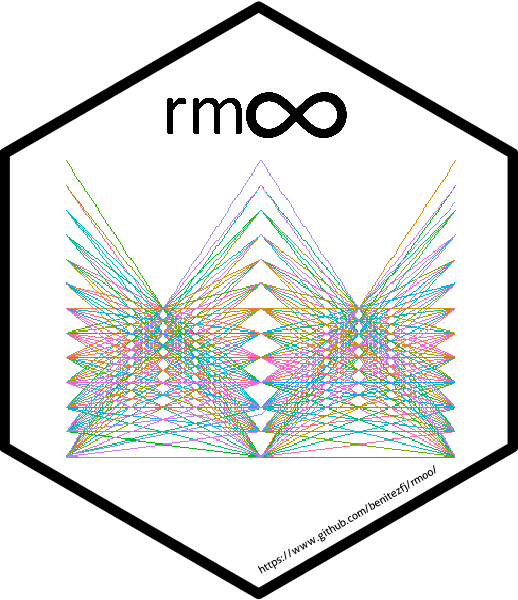# rmoo - R Multi-Objective Optimization## Overview

A Non-Dominated Sorting based Multi-Objective Optimization package, built upon the ‘GA’ package.

`rmoo` provides a complete, flexible and modular framework for optimizing multiple supplied objectives. You will have at your disposal a wide range of configuration options for the NSGA, NSGA-II and NSGA-III algorithms, as well as representation of real numbers, permutations and binaries.

## Installation

You can install the stable version on R CRAN:

``install.packages("rmoo")``

Or you can install the development version from GitHub:

``````# install.packages("devtools")
devtools::install_github("Evolutionary-Optimization-Laboratory/rmoo")``````

## Usage

All the algorithms implemented in `rmoo` are called throught rmoo function. It will receive all the necessary parameters for execution. In this simple example, we solve the DTLZ1 problem, using the NSGA-III:

``````library(rmoo)

DTLZ1 <- function (x, nobj = 3, ...)
{
if (is.null(dim(x))) {
x <- matrix(x, 1)
}
n <- ncol(x)
y <- matrix(x[, 1:(nobj - 1)], nrow(x))
z <- matrix(x[, nobj:n], nrow(x))
g <- 100 * (n - nobj + 1 + rowSums((z - 0.5)^2 - cos(20 *
pi * (z - 0.5))))
tmp <- t(apply(y, 1, cumprod))
tmp <- cbind(t(apply(tmp, 1, rev)), 1)
tmp2 <- cbind(1, t(apply(1 - y, 1, rev)))
f <- tmp * tmp2 * 0.5 * (1 + g)
return(f)
}

result <- rmoo(fitness = DTLZ1,
type = "real-valued",
strategy = "NSGA-III",
lower = c(0,0,0),
upper = c(1,1,1),
monitor = FALSE,
summary = FALSE,
popSize = 92,
n_partitions = 12,
maxiter = 300)``````

The rmoo package has a set of S4 method that can help the user to visualize, analysis, and interpret the results.

We are going to show how use the `plot` method, since depending on the parameter it will display difference plots, e.g. passing “pcp” as parameter for type, the method displays the Parallel Coordinate Plot:

``plot(result, type="pcp")``Another example of plot method is when no parameters are passed, the method by default display the scatter plot. It evaluates the result dimensionality and depending on this, it will display 2-D, 3-D or Pairwise Scatter Plot.

``````#Scatter without optimal points
plot(result)``````When displaying the scatter plot, reference points can be passed as parameters of the optimal argument, allowing the results to be compared to them:

``````#Scatter with optimal points (Using reference points as optimal points)
plot(result, optimal = result@reference_points)``````Other plots available in `rmoo` are heatmap and polar coordinate, both allow the user to view specific solutions.

``````#Polar Coordinates
plot(result, type="polar", individual = c(1:3))````````````#Heatmap Plot
plot(result, type="heatmap", individual = c(1:3))``````Other methods available in `rmoo` that may be of interest are `getFitness()`, `getPopulation()`, `getMetrics()`, `print()`, `summary()` and others. We do not show the functionality of all these functions since they will be detailed in depth in the future article and vignettes.